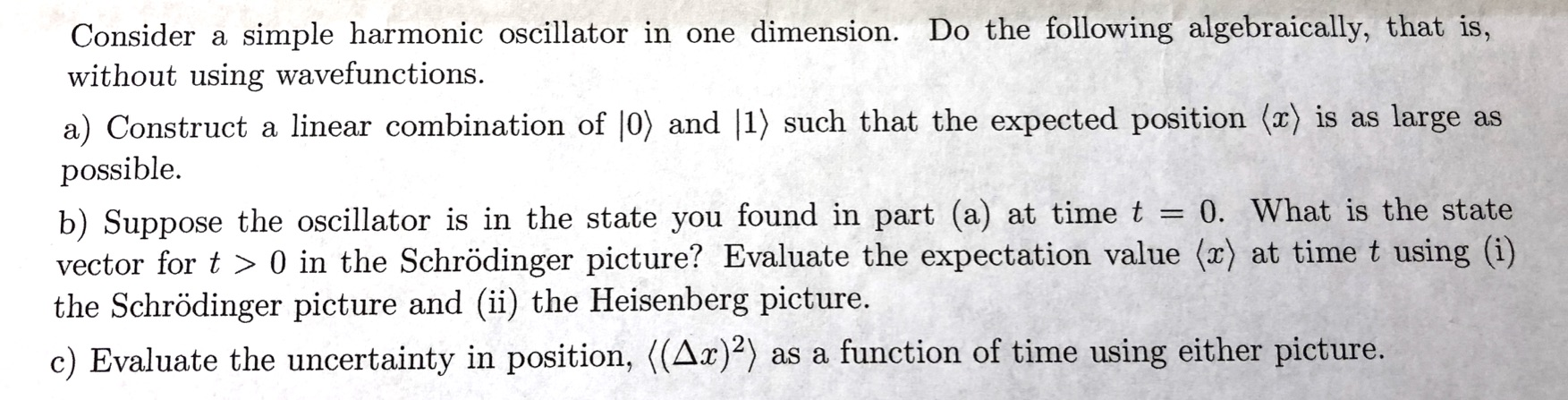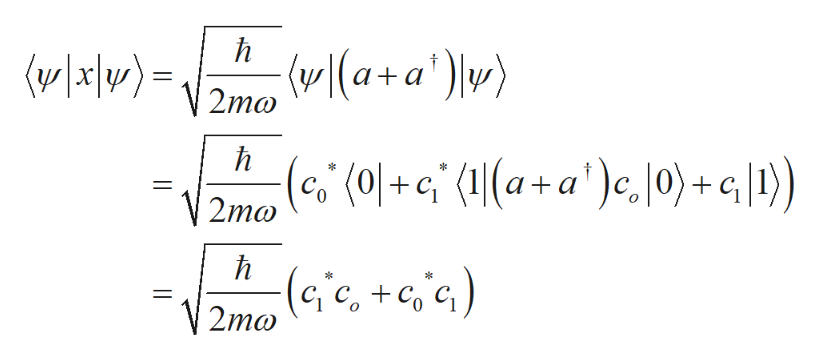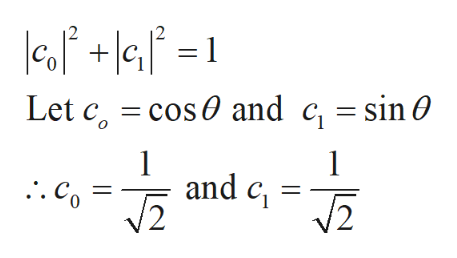# Consider a simple harmonic oscillator in one dimension. Do the following algebraically, that is,without using wavefunctions.a) Construct a linear combination of |0) and |1) such that the expected position (x) is as large aspossibleb) Suppose the oscillator is in the state you found in part (a) at time t -vector for t> 0 in the Schrödinger picture? Evaluate the expectation value (x) at time t using (i)the Schrödinger picture and (ii) the Heisenberg picture.0. What is the statec) Evaluate the uncertainty in position, ((Ax)) as a function of time using either picture.

Question
83 views

It's a quantum mechanics question.help_outlineImage TranscriptioncloseConsider a simple harmonic oscillator in one dimension. Do the following algebraically, that is, without using wavefunctions. a) Construct a linear combination of |0) and |1) such that the expected position (x) is as large as possible b) Suppose the oscillator is in the state you found in part (a) at time t - vector for t> 0 in the Schrödinger picture? Evaluate the expectation value (x) at time t using (i) the Schrödinger picture and (ii) the Heisenberg picture. 0. What is the state c) Evaluate the uncertainty in position, ((Ax)) as a function of time using either picture. fullscreen
check_circle

star
star
star
star
star
1 Rating
Step 1

(a)

The linear combination of the wave function can be written as,

Step 2

The large expectation value of x can be determined as,help_outlineImage Transcriptioncloseh (a+a' w) (vx\v)= V2ma h (01+G (1|(a + a' )e, |0)+G|1) 2ma h (G'c, +c,'c) C 2mo fullscreen
Step 3

To maximize the expec...help_outlineImage Transcriptioncloselesf +le°= cos0 and c = sin 0 Let c, 1 and c . Co fullscreen

### Want to see the full answer?

See Solution

#### Want to see this answer and more?

Solutions are written by subject experts who are available 24/7. Questions are typically answered within 1 hour.*

See Solution
*Response times may vary by subject and question.
Tagged in

### Science